### 【STL 源码剖析】浅谈 STL 迭代器与 traits 编程技法## 前言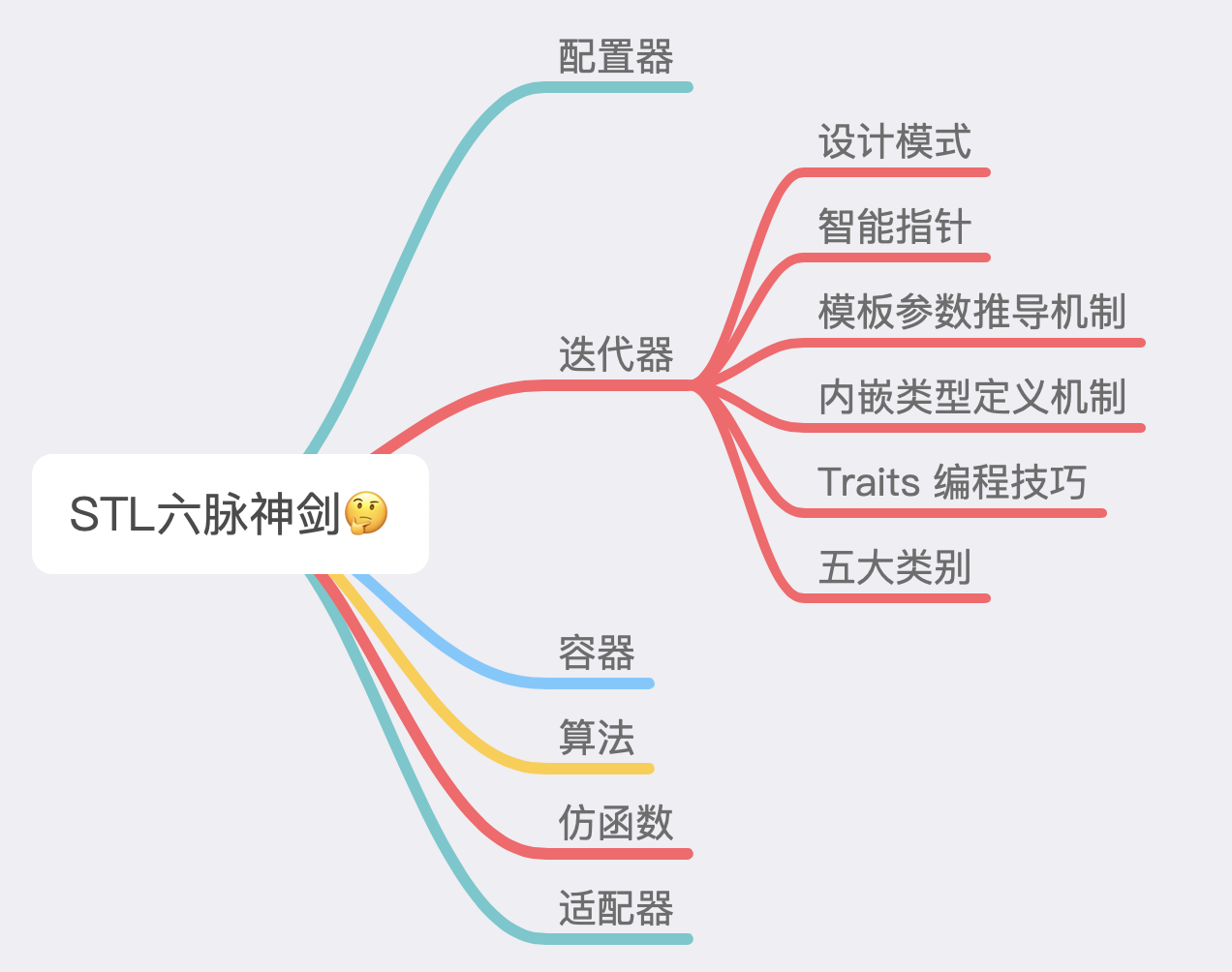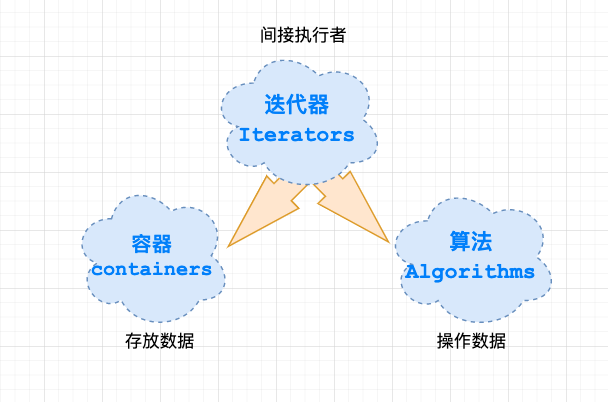## 1、迭代器设计模式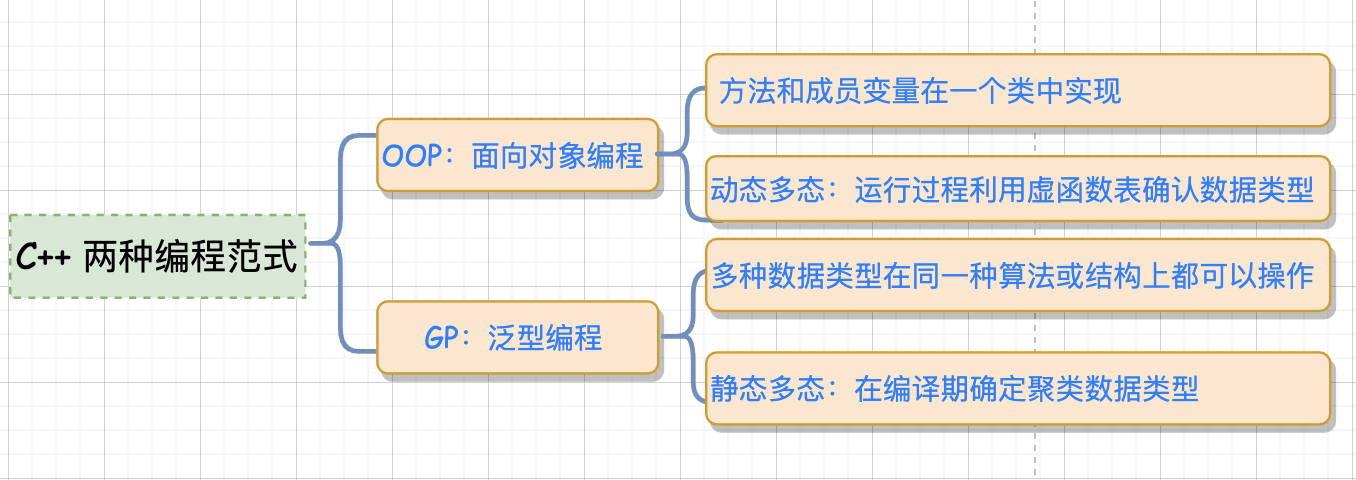OOP：将 methods 和 datas 关联到一起 （通俗点就是方法和成员变量放到一个类中实现），通过继承的方式，利用虚函数表（virtual）来实现运行时类型的判定，也叫”动态多态”，由于运行过程中需根据类型去检索虚函数表，因此效率相对较低。

GP：泛型编程，也被称为”静态多态”，多种数据类型在同一种算法或者结构上皆可操作，其效率与针对某特定数据类型而设计的算法或者结构相同， 具体数据类型在编译期确定，编译器承担更多，代码执行效率高。在 STL 中利用 GP 将 methods 和 datas 实现了分而治之。

## 2、智能指针

STL 是泛型编程思想的产物，是以泛型编程为指导而产生的。具体来说，STL 中的迭代器将范型算法 `(find, count, find_if)` 等应用于某个容器中，给算法提供一个访问容器元素的工具，`iterator` 就扮演着这个重要的角色。

``````template<typename T>
class ListIterator {//mylist迭代器
public:
ListIterator(T *p = 0) : m_ptr(p){} //构造函数
T& operator*() const { return *m_ptr;}  //取值，即dereference
T* operator->() const { return m_ptr;} //成员访问，即member access
//...
};
``````

• (1) 不用担心内存泄漏（类似智能指针，析构函数释放内存）；
• (2) 对于 `list` ，取下一个元素不是通过自增而是通过 `next` 指针来取，使用智能指针可以对自增进行重载，从而提供统一接口

## 3、template 参数推导

`function template` 的参数推导机制是一个不错的方法。

``````template <class I>
inline void func(I iter) {
func_imp(iter, *iter); // 传入 iter 和 iter 所指的值，class 自动推导
}
``````

``````template <class I, class T>
void func_imp(I iter, T t) {
T tmp; // 这里就是迭代器所指物的类别
// ... 功能实现
}
int main() {
int i;
func(&i);//这里传入的是一个迭代器（原生指针也是一种迭代器）
}
``````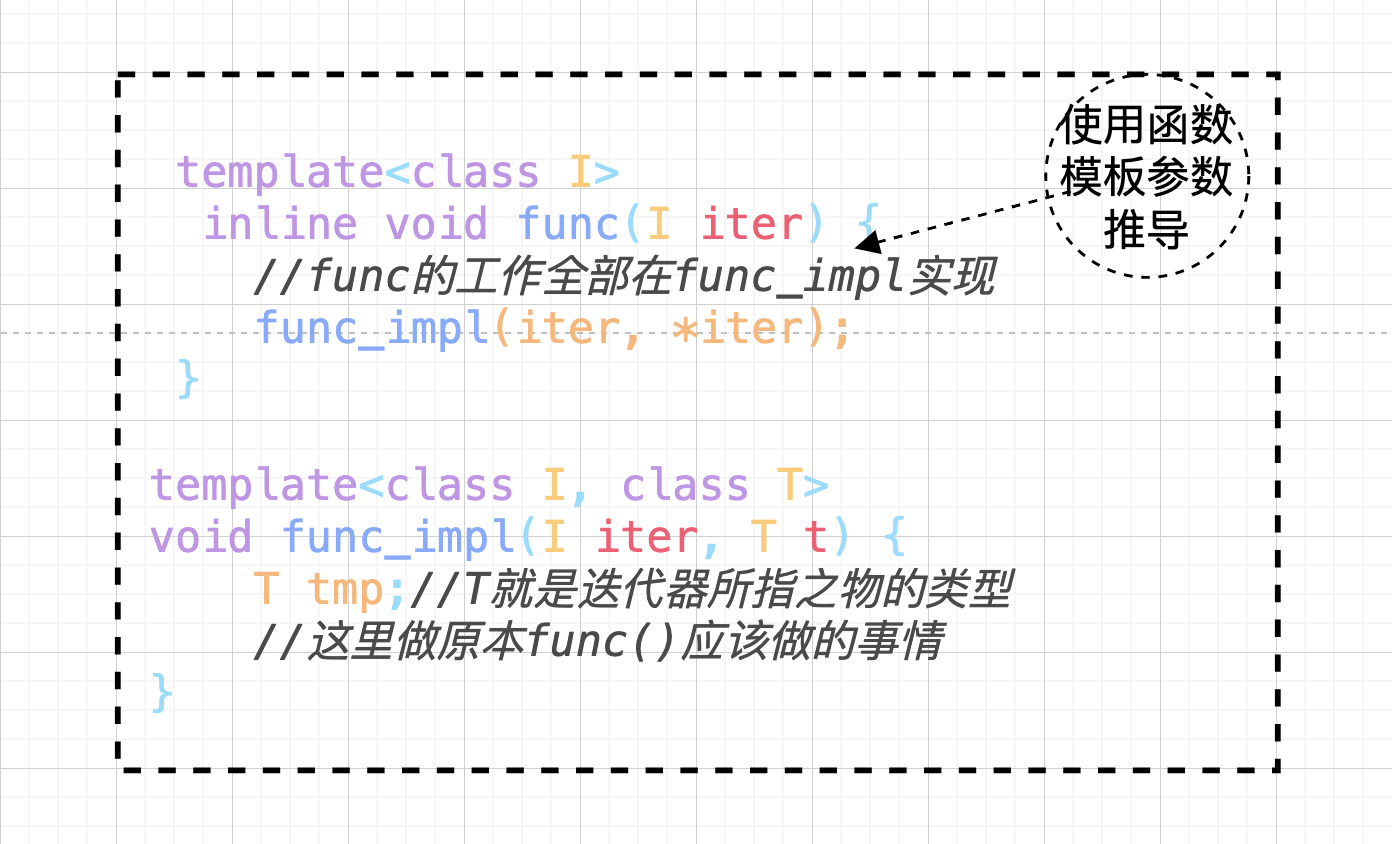## 4、声明内嵌型别

``````template<typename T>
class MyIter {
public:
typedef T value_type; //内嵌类型声明
MyIter(T *p = 0) : m_ptr(p) {}
T& operator*() const { return *m_ptr;}
private:
T *m_ptr;
};

//以迭代器所指对象的类型作为返回类型
//注意typename是必须的，它告诉编译器这是一个类型
template<typename MyIter>
typename MyIter::value_type Func(MyIter iter) {
return *iter;
}

int main(int argc, const  char *argv[]) {
MyIter<int> iter(new int(666));
std::cout<<Func(iter)<<std::endl;  //print=> 666
}
``````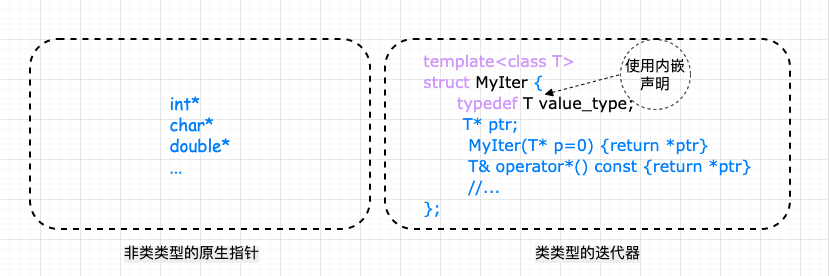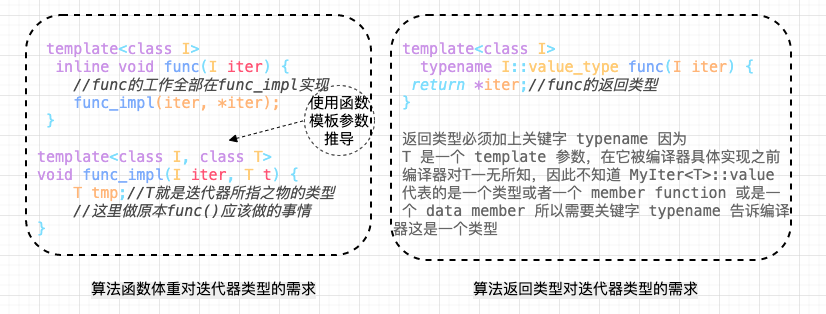``````int *p = new int(5);
cout<<Func(p)<<endl; // error
``````

## 5、Partial specialization（模板偏特化）

``````template <typename T>
class C {...}; //此泛化版本的 T 可以是任何类型
template <typename T>
class C<T*> {...}; //特化版本，仅仅适用于 T 为“原生指针”的情况，是泛化版本的限制版
``````

### 5.1、原生指针怎么办？——特性 “萃取” traits

`traits``bits/stl_iterator_base_types.h` 这个文件中：

``````template<class _Tp>
struct iterator_traits<_Tp*> {
typedef ptrdiff_t difference_type;
typedef typename _Tp::value_type value_type;
typedef typename _Tp::pointer pointer;
typedef typename _Tp::reference reference;
typedef typename _Tp::iterator_category iterator_category;
};
``````
``````template<typename Iterator>
struct iterator_traits {  //类型萃取机
typedef typename Iterator::value_type value_type; //value_type 就是 Iterator 的类型型别
}
``````

``````template<typename Iterator> //萃取前
typename Iterator::value_type  func(Iterator iter) {
return *iter;
}

//通过 iterator_traits 作用后的版本
template<typename Iterator>  //萃取后
typename iterator_traits<Iterator>::value_type  func(Iterator iter) {
return *iter;
}
``````

``````//iterator_traits的偏特化版本，针对迭代器是原生指针的情况
template<typename T>
struct iterator_traits<T*> {
typedef T value_type;
};
``````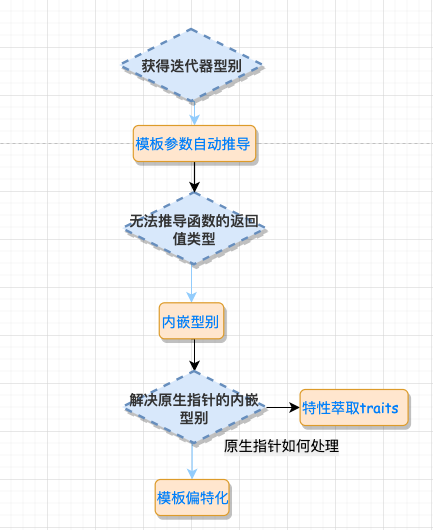### 5.2 、const 偏特化

``````iterator_traits<const int*>::value_type  // 获得的 value_type 是 const int，而不是 int
``````

const 变量只能初始化，而不能赋值（这两个概念必须区分清楚）。这将带来下面的问题：

``````template<typename Iterator>
typename iterator_traits<Iterator>::value_type  func(Iterator iter) {
typename iterator_traits<Iterator>::value_type tmp;
tmp = *iter; // 编译 error
}

int val = 666 ;
const int *p = &val;
func(p); // 这时函数里对 tmp 的赋值都将是不允许的
``````

``````template<typename T>
struct iterator_traits<const T*> { //特化const指针
typedef T value_type; //得到T而不是const T
}
``````

## 6、traits编程技法总结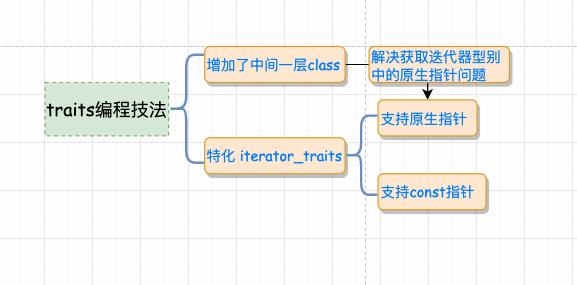``````#include <iostream>

template <class T>
struct MyIter {
typedef T value_type; // 内嵌型别声明
T* ptr;
MyIter(T* p = 0) : ptr(p) {}
T& operator*() const { return *ptr; }
};
// class type
template <class T>
struct my_iterator_traits {
typedef typename T::value_type value_type;
};
// 偏特化 1
template <class T>
struct my_iterator_traits<T*> {
typedef T value_type;
};
// 偏特化 2
template <class T>
struct my_iterator_traits<const T*> {
typedef T value_type;
};

// 首先询问 iterator_traits<I>::value_type,如果传递的 I 为指针,则进入特化版本,iterator_traits 直接回答;如果传递进来的 I 为 class type,就去询问 T::value_type.
template <class I>
typename my_iterator_traits<I>::value_type Func(I ite) {
std::cout << "normal version" << std::endl;
return *ite;
}
int main(int argc, const  char *argv[]) {
MyIter<int> ite(new int(6));
std::cout << Func(ite)<<std::endl;//print=> 6
int *p = new int(7);
std::cout<<Func(p)<<std::endl;//print=> 7
const int k = 8;
std::cout<<Func(&k)<<std::endl;//print=> 8
}
``````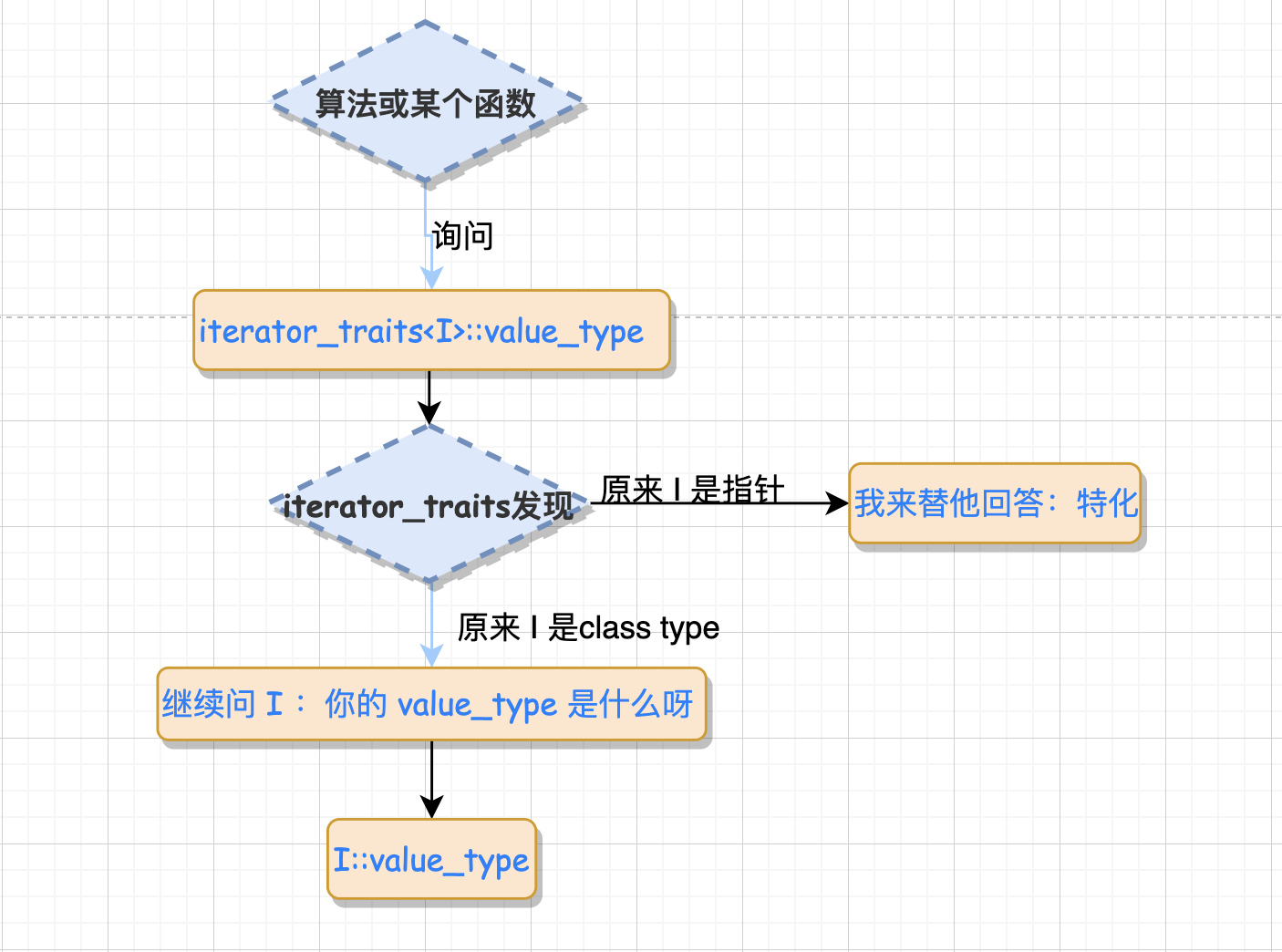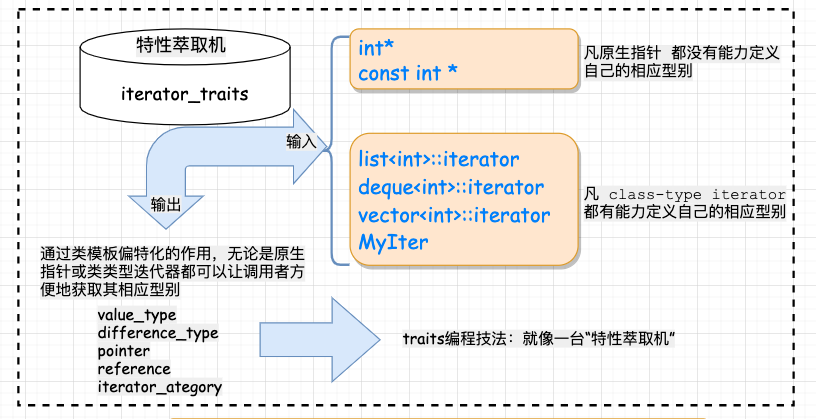## 7、迭代器的型别和种类

#### 7.1 迭代器的型别

• `value_type`：迭代器所指对象的类型，原生指针也是一种迭代器，对于原生指针 int*，int 即为指针所指对象的类型，也就是所谓的 value_type 。

• `difference_type`： 用来表示两个迭代器之间的距离，对于原生指针，STL 以 C++ 内建的 ptrdiff_t 作为原生指针的 difference_type。

• `reference_type`： 是指迭代器所指对象的类型的引用，reference_type 一般用在迭代器的 * 运算符重载上，如果 value_type 是 T，那么对应的 reference_type 就是 T&；如果 value_type 是 const T，那么对应的reference_type 就是 const T&。

• `pointer_type`： 就是相应的指针类型，对于指针来说，最常用的功能就是 operator* 和 operator-> 两个运算符。

• `iterator_category`： 的作用是标识迭代器的移动特性和可以对迭代器执行的操作，从 iterator_category 上，可将迭代器分为 Input Iterator、Output Iterator、Forward Iterator、Bidirectional Iterator、Random Access Iterator 五类，这样分可以尽可能地提高效率。

``````template<typename Category,
typename T,
typename Distance = ptrdiff_t,
typename Pointer = T*,
typename Reference = T&>
struct iterator //迭代器的定义
{
typedef Category iterator_category;
typedef T value_type;
typedef Distance difference_type;
typedef Pointer pointer;
typedef Reference reference;
};
``````

iterator class 不包含任何成员变量，只有类型的定义，因此不会增加额外的负担。由于后面三个类型都有默认值，在继承它的时候，只需要提供前两个参数就可以了。这个类主要是用来继承的，在实现具体的迭代器时，可以继承上面的类，这样子就不会漏掉上面的 5 个型别了

``````tempalte<typename I>
struct iterator_traits {//特性萃取机，萃取迭代器特性
typedef typename I::iterator_category iterator_category;
typedef typename I::value_type value_type;
typedef typeanme I:difference_type difference_type;
typedef typename I::pointer pointer;
typedef typename I::reference reference;
};

//需要对型别为指针和 const 指针设计特化版本看
``````

#### 7.2、迭代器的分类

• `Input Iterator`： 此迭代器不允许修改所指的对象，是只读的。支持 ==、!=、++、*、-> 等操作。
• `Output Iterator`：允许算法在这种迭代器所形成的区间上进行只写操作。支持 ++、* 等操作。
• `Forward Iterator`：允许算法在这种迭代器所形成的区间上进行读写操作，但只能单向移动，每次只能移动一步。支持 Input Iterator 和 Output Iterator 的所有操作。
• `Bidirectional Iterator`：允许算法在这种迭代器所形成的区间上进行读写操作，可双向移动，每次只能移动一步。支持 Forward Iterator 的所有操作，并另外支持 – 操作。
• `Random Access Iterator`：包含指针的所有操作，可进行随机访问，随意移动指定的步数。支持前面四种 Iterator 的所有操作，并另外支持 [n] 操作符等操作。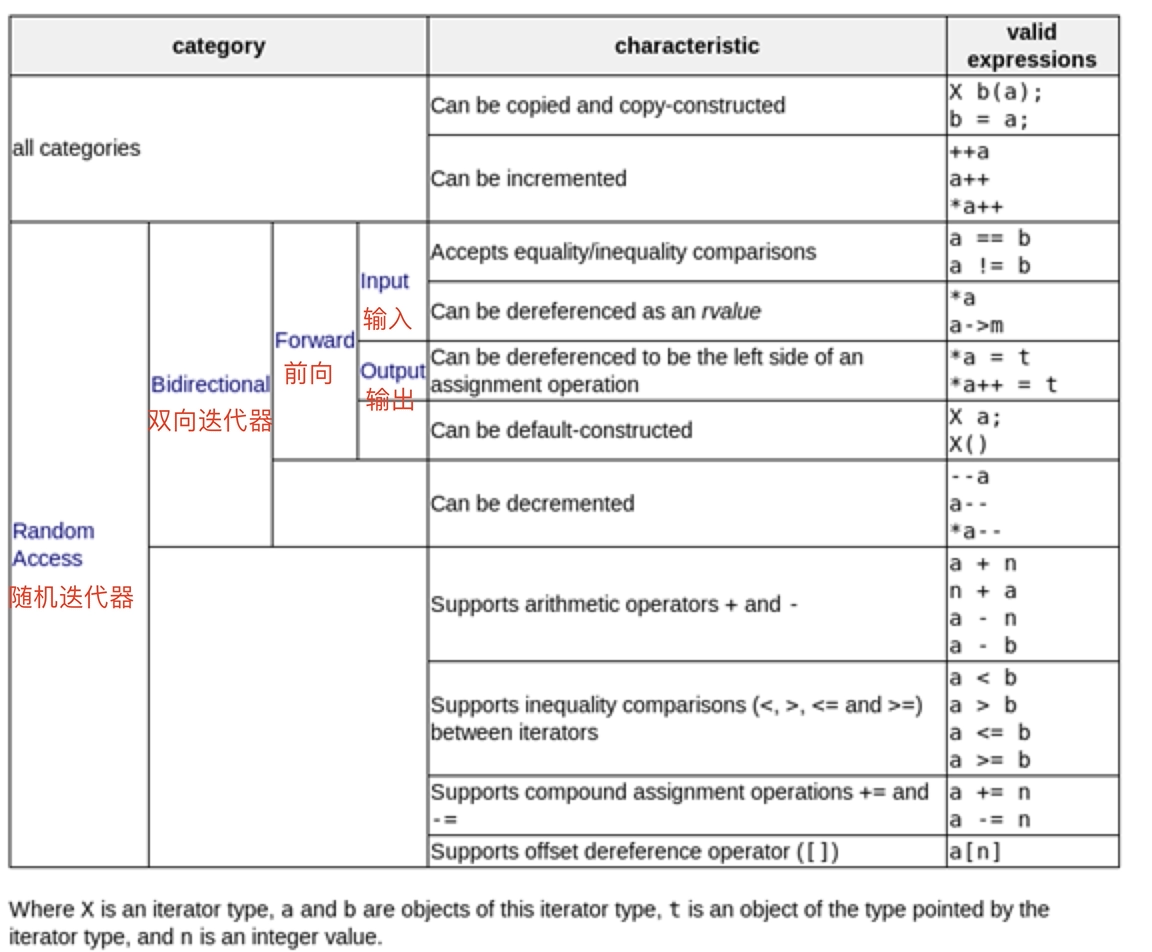Tags: